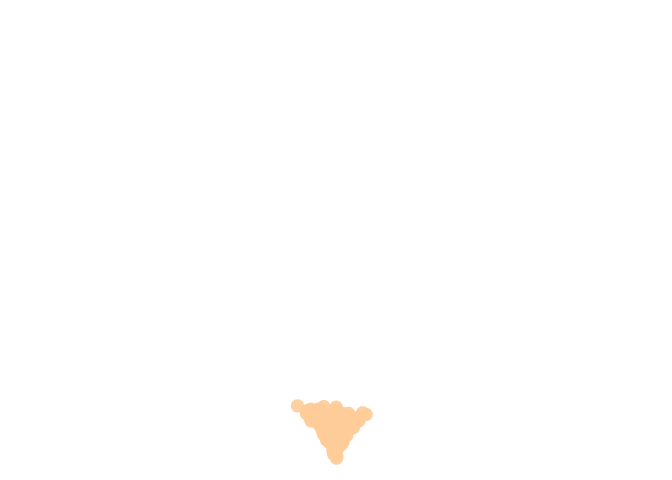# You R My Valentine 2.0!

Like last year I wanted to make something special for my wonderful R-Lady. This year the main work was done by the very talented Will Chase, who makes wonderful aRt including the animation this post is based on. All I did to change the original animation was to cut it into a heart shape and carve our initials into the center. Enjoy:

library(dplyr)
library(poissoned)
library(gganimate)

# generate points
pts <- poisson_disc(ncols = 150, nrows = 400, cell_size = 2,
xinit = 150, yinit = 750, keep_idx = TRUE) %>%
arrange(idx)

# generate heart shape
hrt_dat <- data.frame(t = seq(0, 2 * pi, by = 0.01)) %>%
bind_rows(data.frame(t = rep(max(.$t), 300))) %>% mutate(xhrt = 16 * sin(t) ^ 3, yhrt = 13 * cos(t) - 5 * cos(2 * t) - 2 * cos(3 * t) - cos(4 * t), frame = seq_along(t)) %>% mutate(xhrt = scales::rescale(xhrt, c(min(pts$x), max(pts$x))), yhrt = scales::rescale(yhrt, c(min(pts$y), max(pts$y)))) # identify points within the heart shape inside <- sp::point.in.polygon(point.x = pts$x,
point.y = pts$y, pol.x = hrt_dat$xhrt,
pol.y = hrt_dat$yhrt, mode.checked = FALSE) # animate anim <- pts %>% filter(as.logical(inside)) %>% # cut out heart shape rbind(.[rep(which.min(.$idx), 100), ]) %>% # keep heart visible longer at the end
arrange(idx) %>%
ggplot() +
geom_point(aes(x = x, y = y, color = idx, group = idx), size = 4, alpha = 0.9) +
"#FFA3A3","#FFA699", "#FFB399", "#FFB399",
"#FFC099", "#FFC099","#FFCC99", "#FFCC99"),
guide = "none") +
theme_void() +
geom_text(aes(x = 150, y = 500), label = "A  +  J",
size = 20, colour = "white", family = "Pinyon Script") + # add text
transition_reveal(along = rev(idx)) +
ease_aes("cubic-in") +
enter_grow() +
animate(anim, nframes = 300, fps = 30)